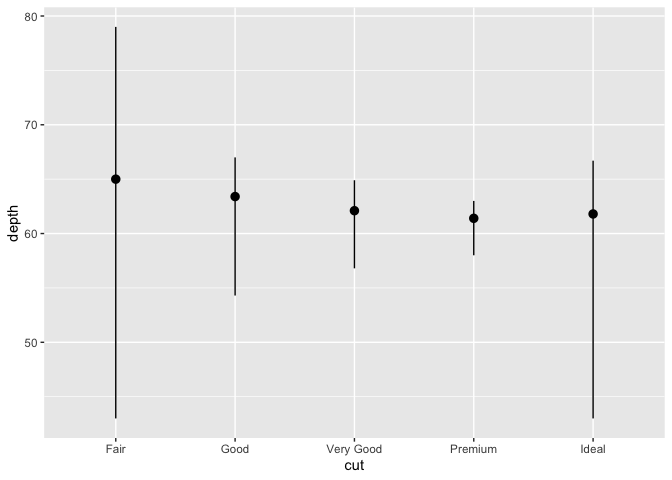# Statistical Transformation in ggplot2

## Statistical Transformations

• Before we discuss transformation in graphs, we will take a quick look at a bar chart.
library(tidyverse)
ggplot(data = diamonds) +
geom_bar(mapping = aes(x = cut))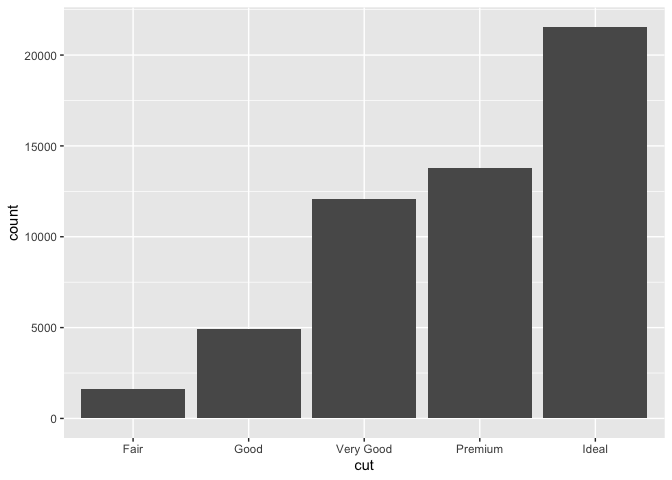• The above bar chart displays the total number of diamonds in the diamonds dataset, grouped by cut.
• geom_bar only requires one aesthetic, that is x.
• On the y-axis, it displays count. But count is not a variable in diamonds!
• Many plots (like scatterplot) the raw values of your dataset.
• Other graphs (like bar charts, histograms, etc.) calculate new values to plot:
• bar charts, histograms, and frequency polygons bin your data and then plot bin counts, the number of points that fall in each bin.
• smoothers fit a model to your data and then plot predictions from the model.
• boxplots compute a robust summary of the distribution and then display a specially formatted box.
• The algorithm used to calculate new values for a graph is called a stat, short for statistical transformation.

### geom and stat functions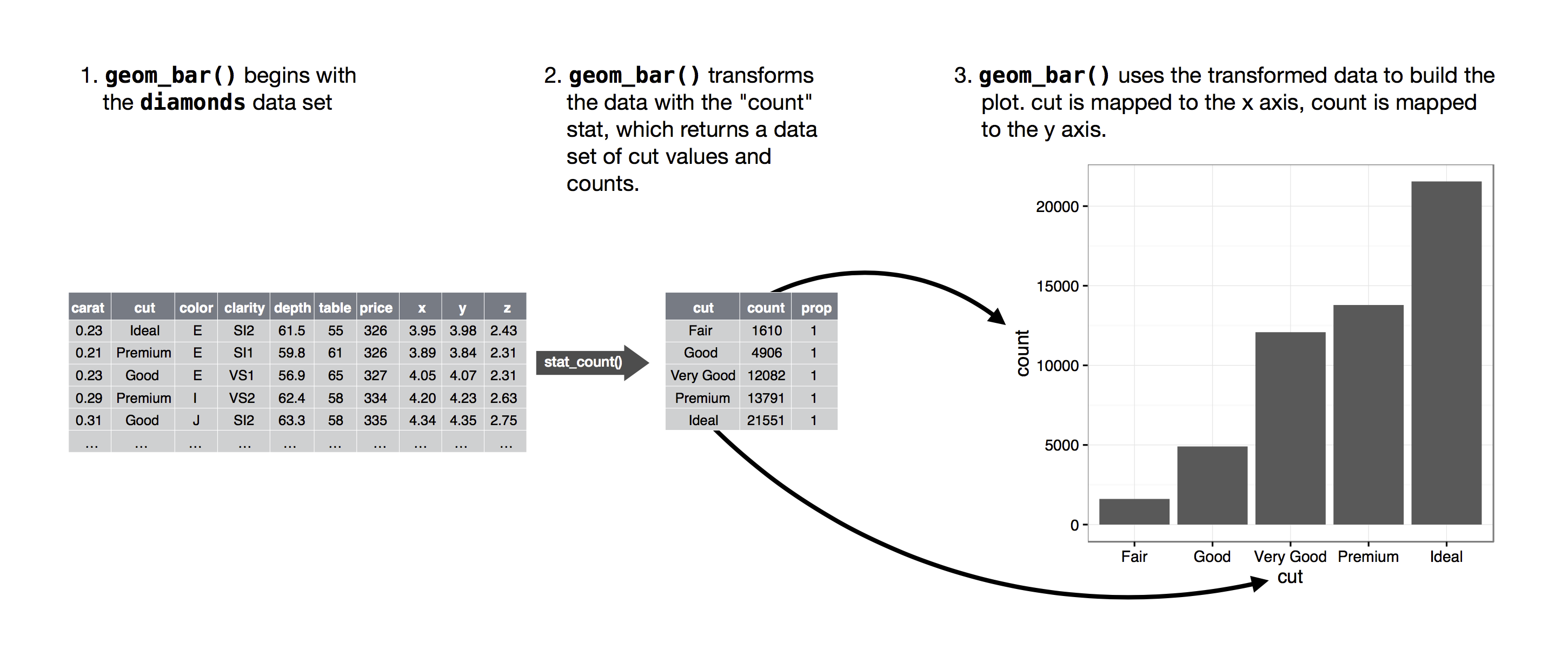• You can generally use geoms and stats interchangeably. For example, you can recreate the previous plot using stat_count() instead of geom_bar():
ggplot(data = diamonds) +
stat_count(mapping = aes(x = cut))• This works because every geom has a default stat; and every stat has a default geom.

### Use a specific stat for graphing

There are three reasons you might need to use a stat explicitly:

• You might want to override the default stat.
demo <- tribble(
~cut,         ~freq,
"Fair",       1610,
"Good",       4906,
"Very Good",  12082,
"Premium",    13791,
"Ideal",      21551
)

ggplot(data = demo) +
geom_bar(mapping = aes(x = cut, y = freq), stat = "identity")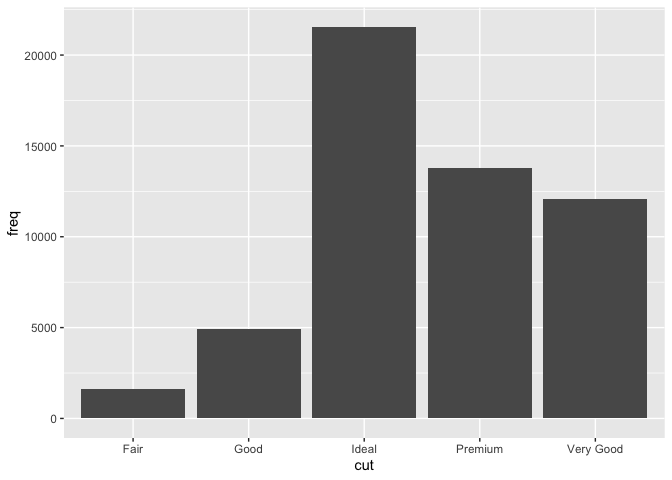• You might want to override the default mapping from transformed variables to aesthetics.
• For example, you might want to display a bar chart of proportion, rather than count:
ggplot(data = diamonds) +
geom_bar(mapping = aes(x = cut, y = stat(prop), group = 1))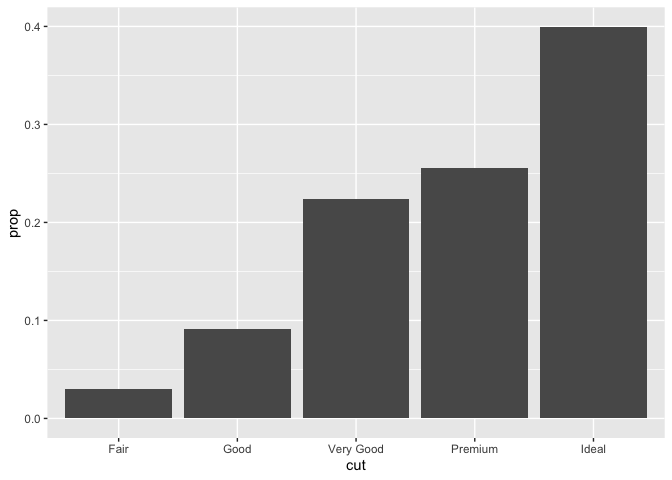• You might want to draw greater attention to the statistical transformation in your code.
• For example, you might use stat_summary(), which summarises the y values for each unique x value, to draw attention to the summary that you’re computing:
ggplot(data = diamonds) +
stat_summary(
mapping = aes(x = cut, y = depth),
fun.ymin = min,
fun.ymax = max,
fun.y = median
)